# Mapping and analyzing road accidents in South Australia

## 23 Jan 2018

Since I may already have acquired a bit of a reputation for being preoccupied with accident analyses, I decided to give it another shot and map and analyze all (non-)fatal road accidents happening in South Australia in 2016.

Data for this analysis is provided by the South Australian Government Data Directory.

## Setup

Running the code below requires the packages `ggmap`, `ggplot2`, `ggthemes`, `magrittr`, `maps`, `mapdata`, `maptools`, `proj4`, `raster`, `rgdal`, `sp`, `stringr`, and `tidyverse`.

For loading multiple packages at once, I conventionally use `p_load()` from the `pacman` package which is a wrapper function for `library()` and `require()` and installs missing packages if necessary.

``````# Install and load pacman if not already installed
if (!require("pacman")) install.packages("pacman")
library(pacman)

p_load(ggmap, ggplot2, ggthemes, magrittr, maps, mapdata, maptools, proj4, raster, rgdal, sp, stringr, tidyverse)
``````

To download the data for the subsequent analysis, either go to the South Australian Government Data Directory’s website and download `road-crashes-in-sa-2014-16.zip` manually, unzip it and import `2016_DATA_SA_Crash.csv` or run this code directly in `R`:

``````# Download, unzip and import data on road crashes in 2016
temp <- tempfile()

unzip(temp)

``````

After getting the data into `R`, some minor data cleaning is required.

``````# Replace blank spaces in column names with underscores and convert letters to lowercase
colnames(accidents) <- gsub(" ", "_", str_trim(tolower(names(accidents))), fixed = TRUE)

# Add column for fatality status of each crash (fatal/non-fatal)
accidents\$fatality <- NA # prevents tibble error message
accidents\$fatality[accidents\$csef_severity == "4: Fatal"] <- "Fatal"
accidents\$fatality[accidents\$csef_severity != "4: Fatal"] <- "Non-fatal"

# Recode type of crash
``````

## Spatial data wrangling

Prior to finally analyzing the accident data, some more geographical data wrangling needs to be done.

As a first step we need to choose the right projection for transforming the `x` and `y` coordinates provided in the data set into their respective `longitude` and `latitude` equivalents.

The data itself comes with the following information on the projection (EPSG:3107)

``````PROJECTION LAMBERT
UNITS METERS
DATUM GDA94 SEVEN /* GDA94 SPHEROID GRS80 PARAMETERS
-28 00 00 /* 1st standard parallel
-36 00 00 /* 2nd standard parallel
135 00 00 /* Central meridian
-32 00 00 /* Latitude of projections origin
1000000 /* False easting (meters)
2000000 /* False northin (meters)
END
``````

which translates into this `Proj4 string` (thanks to the people over at GIS Stack Exchange for helping me out on this one):

``````# Projection for accident coordinates (EPSG:3107)
proj <- "+proj=lcc +lat_1=-28 +lat_2=-36 +lat_0=-32 +lon_0=135 +x_0=1000000 +y_0=2000000 +ellps=GRS80 +towgs84=0,0,0,0,0,0,0 +units=m +no_defs"
``````

After setting the right projection/coordinate reference system, we can then transform the accident coordinates to spatial points using `project()` from the `proj4` package.

``````# Code spatial points and convert to data frame
points <- proj4::project(accidents[, c("accloc_x", "accloc_y")], proj = proj, inverse = TRUE)

accidents\$longitude <- points\$x
accidents\$latitude <- points\$y

names(accidents)[names(accidents) == "longitude"] <- "long"
names(accidents)[names(accidents) == "latitude"] <- "lat"

accidents_df <- as.data.frame(accidents)
``````

In order to map the spatial points we need matching Australian shapefiles (`.shp`; a geospatial vector data format for `GIS` software), which luckily can be found at the South Australian Government Data Directory’s website as well and consist of both country outlines and state borders.

To obtain the shapefiles, download `nsaasr9nnd_02211a04es_geo___.zip`, unzip it and load `aust_cd66states.shp` into `R` or, again, use the automated code provided below:

``````# Download, unzip and import Australian shapefiles

temp <- tempfile()

unzip(temp)

aus_shp <- readShapeSpatial("aust_cd66states.shp", proj4string = CRS("+proj=longlat +ellps=WGS84"))

``````

For creating a tailor-made map depicting road accidents in South Australia only, we need to subset the state polygon from the spatial `SpatialPolygonsDataFrame` object `aus_shp` (`STE` denotes state).

``````# Subset South Australia
sa_shp <- subset(aus_shp, STE == 4)
``````

While `ggplot2` maps are nice in themselves, inspired by this post, we attempt to make them even nicer by modifying the basic theme in `theme_map()` as follows

``````# Set theme for maps
map_theme <- theme_map(base_family = "Avenir") +
theme(strip.background = element_blank(),
strip.text = element_text(size = rel(1), face = "bold"),
text = element_text(size = 10),
plot.caption = element_text(colour = "grey50"))
``````

and plot the first map showing all fatal and non-fatal road crashes in South Australia in 2016. (Note that several points lie outside the polygon.)

``````# Plot road accidents
ggplot() +
geom_polygon(data = aus_shp, aes(x = long, y = lat, group = group)) +
geom_point(data = accidents_df, aes(x = long, y = lat, colour = fatality), size = 1) +
labs(x = "", y = "", title = "Road crashes in South Australia, 2016", subtitle = "(fatal and non-fatal crashes),
caption = "Data source: South Australian Government Data Directory"") + map_theme +
scale_color_manual(name = "Severity", values = c("Fatal" = "red2", "Non-fatal" = "grey")) +
coord_equal()
``````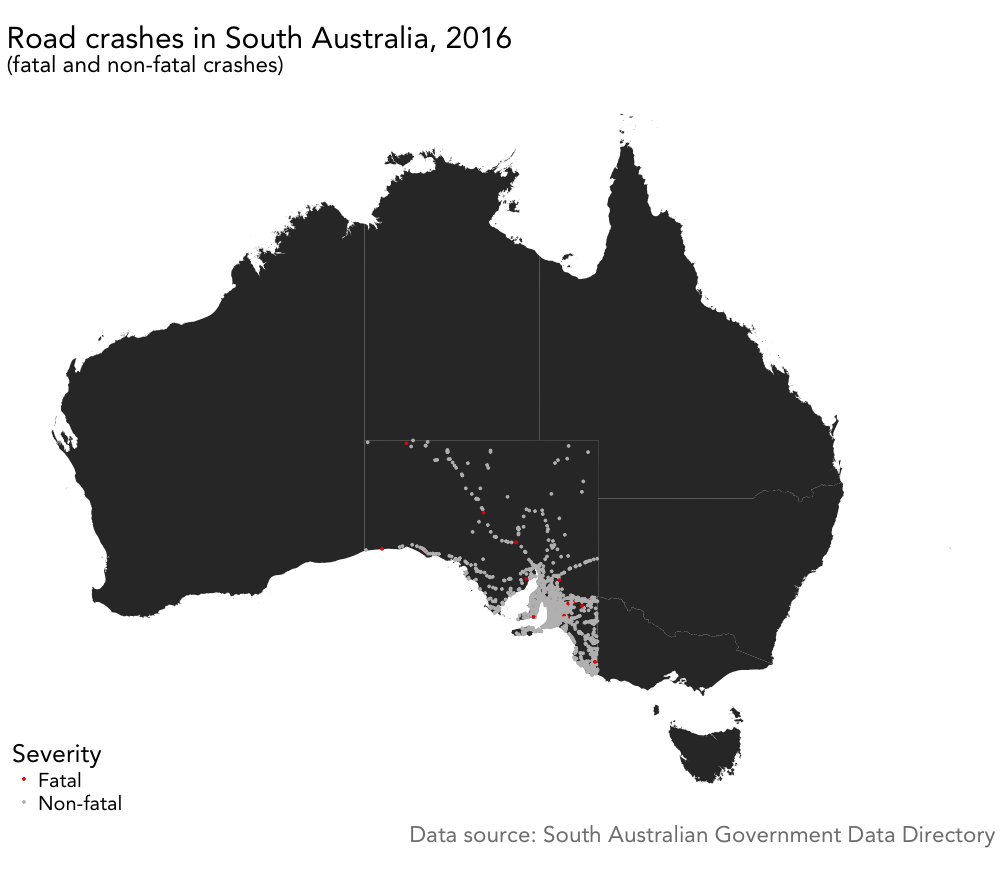Since the accident data at hand only covers crashes in one particular state, we use the subsetted `sa_shp` polygon to restrict the map to the South Australian area

``````# Plot road accidents with point sizes adjusted by number of casualties
ggplot() +
geom_polygon(data = sa_shp, aes(x = long, y = lat, group = group)) +
geom_point(data = accidents_df, aes(x = long, y = lat, colour = fatality, size = total_cas/1.5)) +
labs(x = "", y = "", title = "Road crashes in South Australia, 2016", subtitle = "(by severity and number of casualties)") + map_theme +
scale_color_manual(name = "Severity", values = c("Fatal" = "red2", "Non-fatal" = "grey")) +
scale_size_identity() + coord_equal()
``````

which yields the following map, where the point sizes reflect the total number of casualties (divided by 1.5 for aesthetic reasons):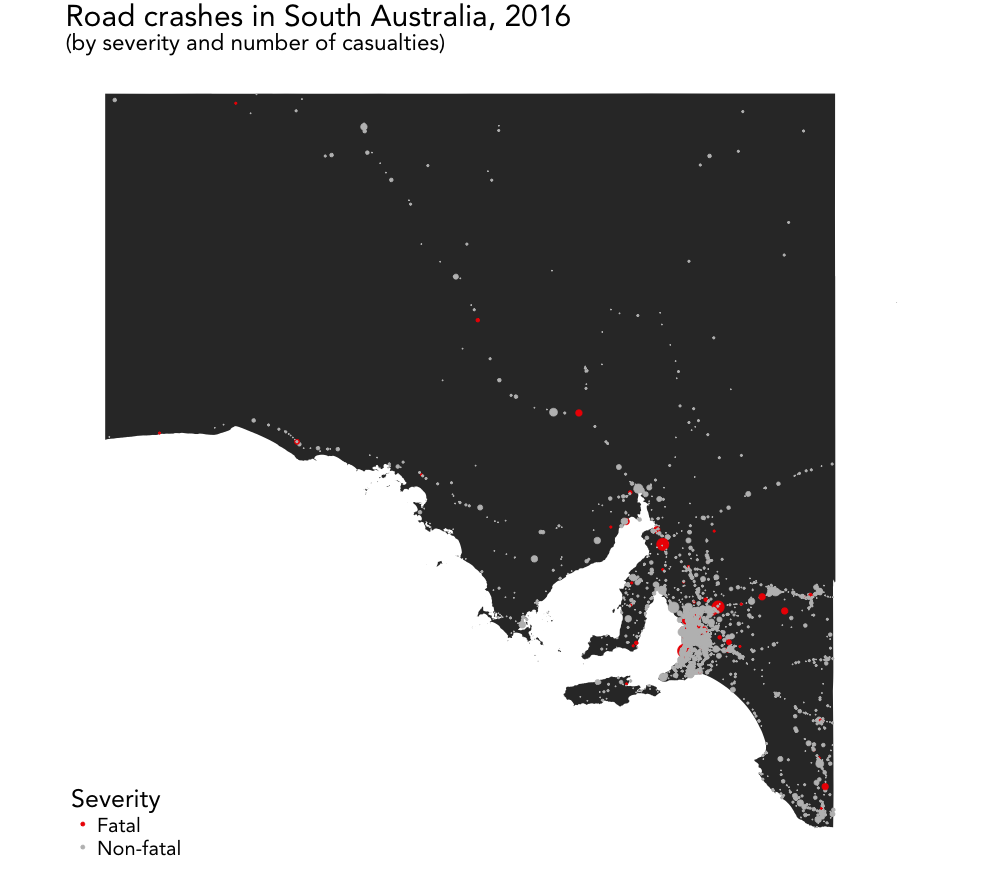## Visualizing crashes by type

After having mapped all road crashes, we can further analyze the data by visualizing some basic statistics.

As before, we customize the theme of the `ggplot2` map using `theme()` to somewhat match the layout of the map we previously built

``````# Set theme for visualizations
viz_theme <- theme(
strip.background = element_blank(),
strip.text = element_text(size = rel(1), face = "bold"),
plot.caption = element_text(colour = "grey50"),
axis.text.x = element_text(angle = 65, vjust = 0.5),
text = element_text(family = "Avenir"))
``````

and make a nice looking graph of the total number of crashes by crash type and severity of crash (fatal/non-fatal) by running

``````# Plot number of crashes by crash type and severity
ggplot(accidents_df, aes(crash_type)) +
geom_bar(aes(fill = fatality), width = 0.8) +
labs(x = "", y = "", title = "Number of crashes by crash type and severity", subtitle = "South Australia, 2016") +
scale_fill_manual(name = "Severity", values = c("Fatal" = "red2", "Non-fatal" = "grey35")) +
viz_theme + ylim(0, 5000) # + theme(legend.position = "bottom")
``````

and thus creating this plot: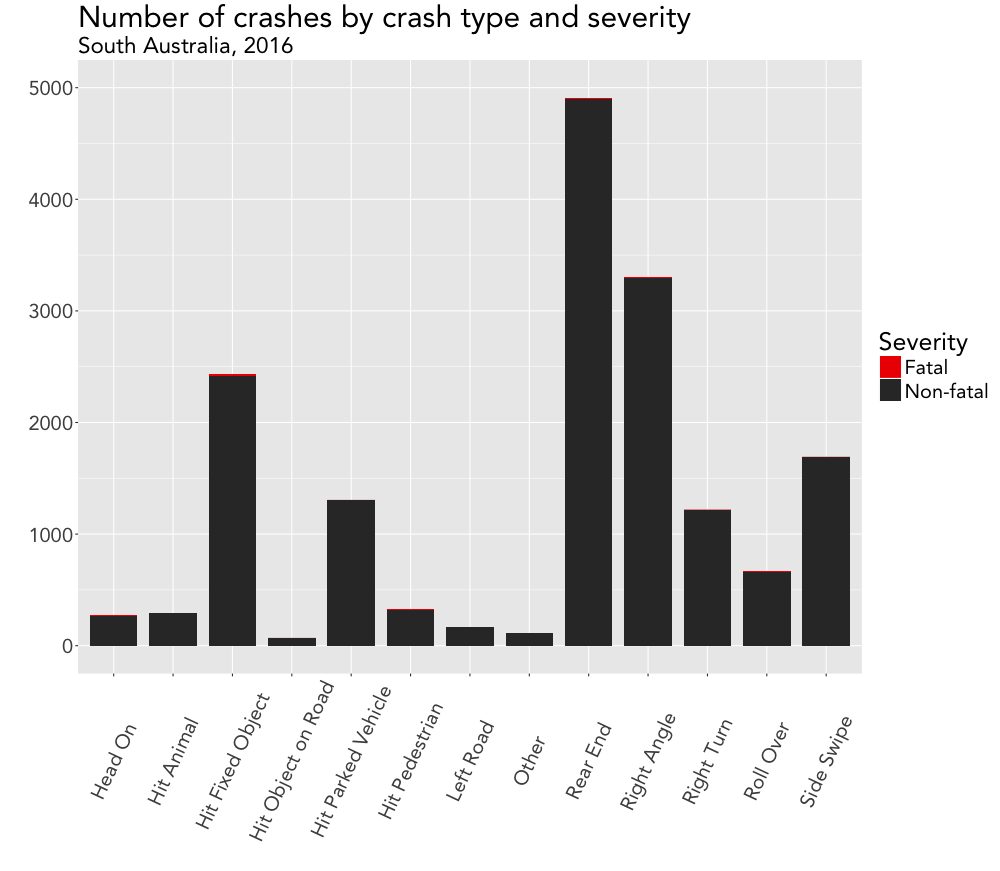## Visualizing casualties by crash type

In a similar manner, we can make another bar chart for the number of casualties by crash type. Prior to plotting, however, we need to calculate the corresponding numbers first.

As a bonus feature we now rearrange the bars of the plot by ordering the crash types by number of casualties in ascending order.

``````# Calculate total number of casualties by crash type
accidents_cas <- accidents_df[, c("crash_type", "total_cas")]

accidents_cas %<>%
group_by(crash_type) %>%
summarise(cas_sum = sum(total_cas))
names(accidents_cas) <- c("crash_type", "total_cas")

# Order crash types by number of casualties
accidents_cas_ordered <- accidents_cas %>%
arrange(total_cas, crash_type) %>%
mutate(crash_type = factor(crash_type, unique(crash_type)))

# Plot number of casualties by crash type (ordered)
ggplot(accidents_cas_ordered, aes(crash_type, total_cas)) +
geom_bar(stat = "identity", width = 0.8, color = "red", fill = "black") +
labs(x = "", y = "", title = "Number of casualties (fatal and non-fatal) by crash type", subtitle = "South Australia, 2016") +
viz_theme + ylim(0, 2000)
``````

These three chunks of code then produce the following plot: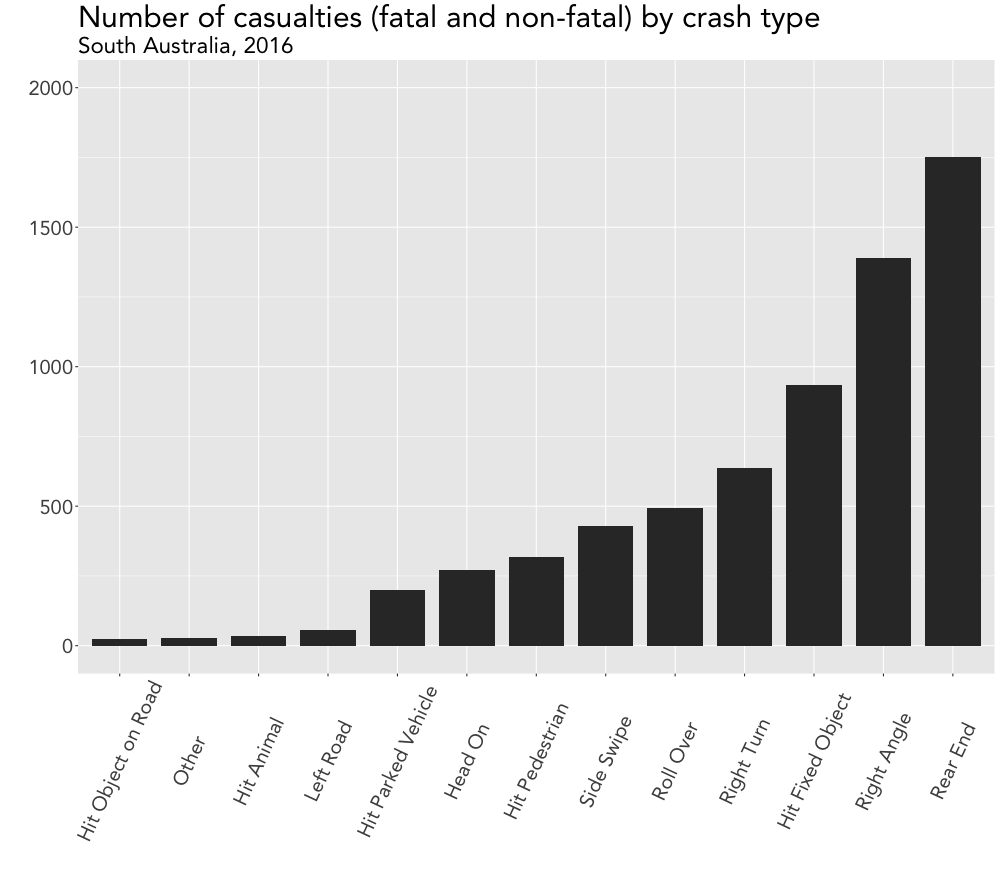## Bonus: Visualizing casualties via lollipop chart

While browsing this site, I found both inspiration and sample codes for making some #dataviz magic happen.

As a result, I decided to finish up this post by complementing the previous chart with its more modern looking lollipop counterpart.

``````# Lollipop chart of number of casualties by crash type
ggplot(accidents_cas, aes(x = crash_type, y = total_cas)) +
geom_point(size = 3, color = "red", fill = "grey35") +
geom_segment(aes(x = crash_type,
xend = crash_type,
y = 0,
yend = total_cas)) +
labs(x = "", y = "", title = "Number of casualties (fatal and non-fatal) by crash type", subtitle = "South Australia, 2016") +
viz_theme + ylim(0, 2000)
``````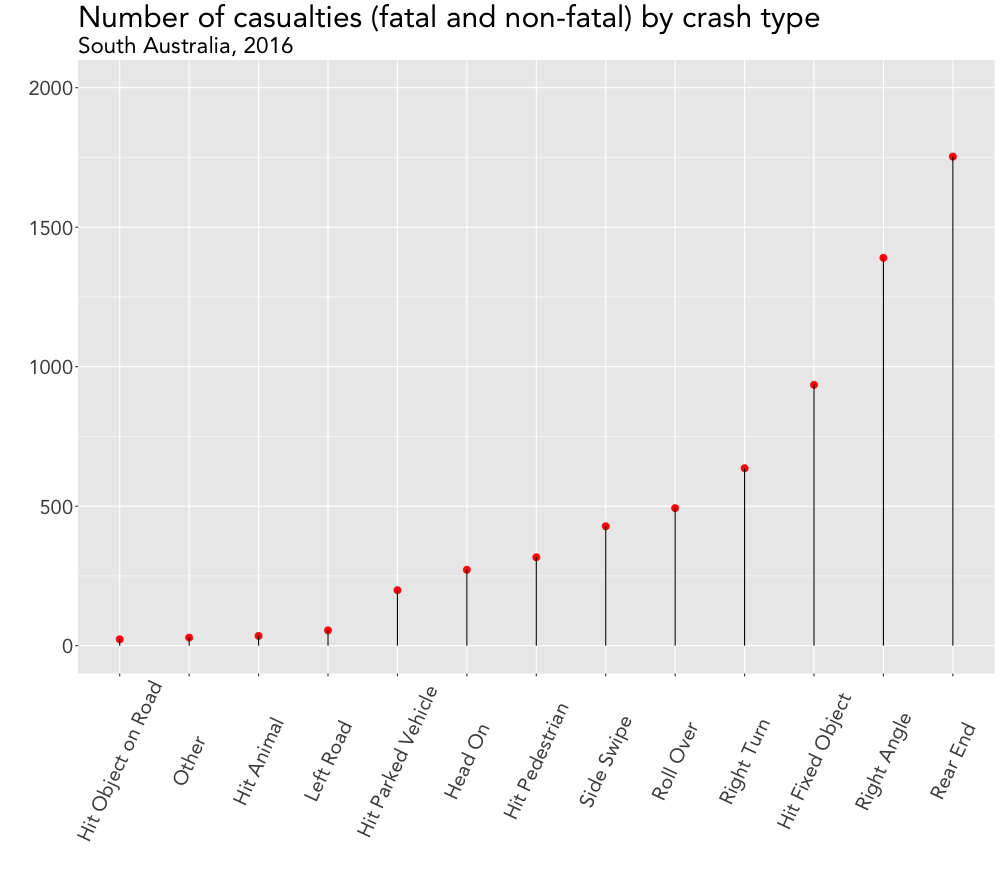Note: The above code is only a (slightly adapted) snippet of the full script, which can be found here.